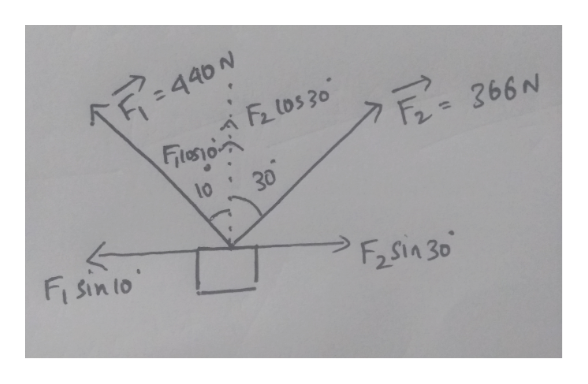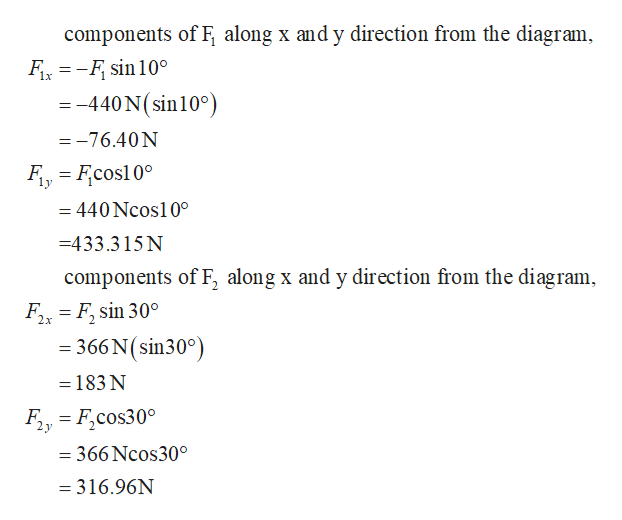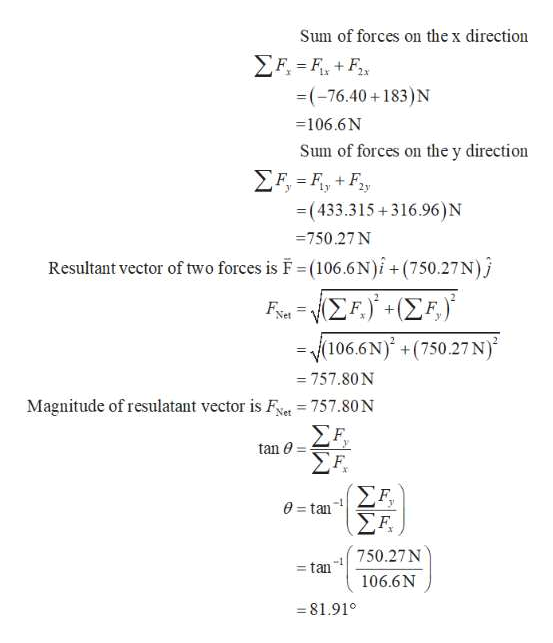An illustration shows an overhead view of a car as it approaches a fork in the road. The car is oriented such that it is directed toward the top of the illustration. A force F1 extends from the nose of the car and points along the left-side forked road at an angle of 10° measured counterclockwise from the car's initial direction. A force F2 extends from the nose of the car and points along the right-side forked road at an angle of 30° measured clockwise from the car's initial direction. Two forces are applied to a car in an effort to move it, as shown in the figure below. (Let F1 = 440 N and F2 = 366 N. Assume up and to the right are in the positive directions.) (a) What is the resultant vector of these two forces? (b) If the car has a mass of 3,000 kg, what acceleration does it have? Ignore friction.

Question
An illustration shows an overhead view of a car as it approaches a fork in the road. The car is oriented such that it is directed toward the top of the illustration. A force F1 extends from the nose of the car and points along the left-side forked road at an angle of 10° measured counterclockwise from the car's initial direction. A force F2 extends from the nose of the car and points along the right-side forked road at an angle of 30° measured clockwise from the car's initial direction.

Two forces are applied to a car in an effort to move it, as shown in the figure below. (Let
F1 = 440 N
and
F2 = 366 N.
Assume up and to the right are in the positive directions.)

(a) What is the resultant vector of these two forces?

(b) If the car has a mass of 3,000 kg, what acceleration does it have? Ignore friction.
Step 1

Draw the components of the forces F1  and F2.help_outlineImage Transcriptionclose=440N FLOS 30 Fieio 366N 10 30 Fi sin to F2sin 30 fullscreen
Step 2

Write the x and y components of the force F1  and F2 from the diagram.help_outlineImage Transcriptionclosecomponents of F along x and y direction from the diagram, FR =-F sin10° = -440 N (sin10°) =-76.40 N FFcos10 1у - 440 Ncos10° -433.315 N components of F, along x and y direction from the diagram Fir F sin 30° - 366 N (sin30°) 2x = 183 N Fy F,cos30 = 366 Ncos30° = 316.96N fullscreen
Step 3

Continuing previ...help_outlineImage TranscriptioncloseSum of forces on the x direction FFF =(-76.40+183)N 106.6N Sum of forces on the y direction F FFy + -(433.315+316.96)N = 750.27 N Resultant vector of two forces is F (106.6N)i +(750.27 N) E.-Σ.)- Ση Net (106.6N) +(750.27N) 757.80 N Magnitude of resulatant vector is Fet 757.80 N Σ . Ση tan Σ . ΣΗ 0=tan 750.27N - tan 106.6N 81.91° WW fullscreen

Want to see the full answer?

See Solution

Want to see this answer and more?

Our solutions are written by experts, many with advanced degrees, and available 24/7

See Solution
Tagged in

Newtons Laws of Motion